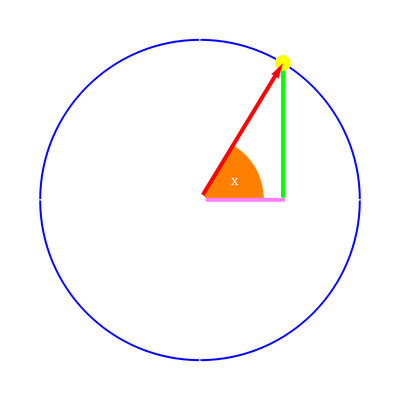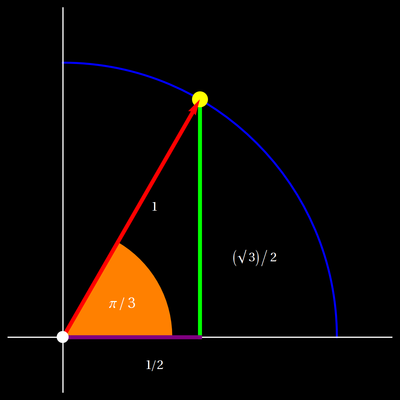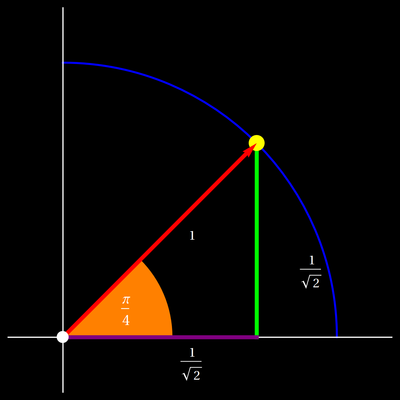# The Cos and Sin functionsThe picture to the left is very important. You can read off the values of sin(x) = opposite side/hypothenuse, cos(x) = adjacent side/hypothenuse. as well as tan(x) = opposite side/adjacent side, which is the slope. Here are the formulas you need to know ```sin2(x) + cos2(x) = 1 sin(2x) = 2 sin(x) cos(x) cos(2x) = cos2(x)-sin2(x) ``` Exhibit of 2011 with animation Additionally, we need to know the following two triangles.